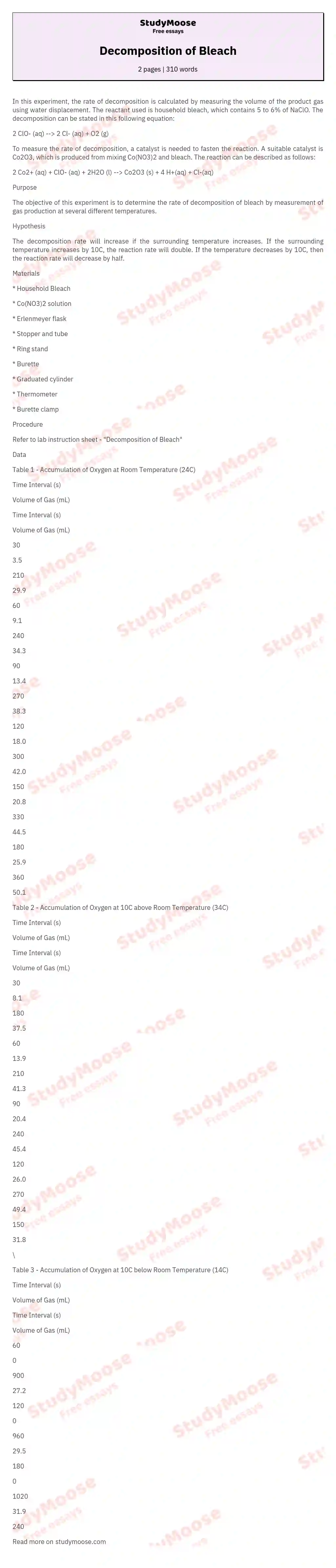# Decomposition of Bleach

In this experiment, the rate of decomposition is calculated by measuring the volume of the product gas using water displacement. The reactant used is household bleach, which contains 5 to 6% of NaClO. The decomposition can be stated in this following equation:

2 ClO- (aq) –> 2 Cl- (aq) + O2 (g)

To measure the rate of decomposition, a catalyst is needed to fasten the reaction. A suitable catalyst is Co2O3, which is produced from mixing Co(NO3)2 and bleach. The reaction can be described as follows:

2 Co2+ (aq) + ClO- (aq) + 2H2O (l) –> Co2O3 (s) + 4 H+(aq) + Cl-(aq)

Purpose

The objective of this experiment is to determine the rate of decomposition of bleach by measurement of gas production at several different temperatures.

Hypothesis

The decomposition rate will increase if the surrounding temperature increases. If the surrounding temperature increases by 10C, the reaction rate will double. If the temperature decreases by 10C, then the reaction rate will decrease by half.

Materials

* Household Bleach

* Co(NO3)2 solution

* Stopper and tube

* Ring stand

* Burette

* Thermometer

* Burette clamp

Procedure

Refer to lab instruction sheet – “Decomposition of Bleach”

Data

Table 1 – Accumulation of Oxygen at Room Temperature (24C)

Time Interval (s)

Volume of Gas (mL)

Time Interval (s)

Volume of Gas (mL)

30

3.5

210

29.9

60

9.1

240

34.3

90

13.4

270

38.3

120

18.0

300

42.0

150

20.8

330

44.5

180

25.9

360

50.1

Table 2 – Accumulation of Oxygen at 10C above Room Temperature (34C)

Time Interval (s)

Volume of Gas (mL)

Time Interval (s)

Volume of Gas (mL)

30

8.1

180

37.5

60

13.9

210

41.3

90

20.4

240

45.4

120

26.0

270

49.4

150

31.8

\

Table 3 – Accumulation of Oxygen at 10C below Room Temperature (14C)

Time Interval (s)

Volume of Gas (mL)

Time Interval (s)

Volume of Gas (mL)

60

0

900

27.2

120

0

960

29.5

180

0

1020

31.9

240

1.8

1080

33.5

300

4.0

1140

36.0

360

6.5

1200

38.0

420

8.9

1260

40.5

540

11.8

1320

42.1

600

13.2

1380

44.1

660

15.6

1440

45.6

720

18.1

1500

47.5

780

21.0

1560

49.6

840

23.0

1579

50.0

Analysis

Calculations:

Reaction Rate =

Reaction rate at room temperature = = 0.14 mL O2 / s

Reaction rate at 10C above room temperature = = 0.17 mL O2 / s

Reaction rate at 10C below room temperature = = 0.036 mL O2 / s

Table 4 – Rate of Decomposition of Bleach

Surrounding Temperature (C)

Reaction Rate (mL/ s)